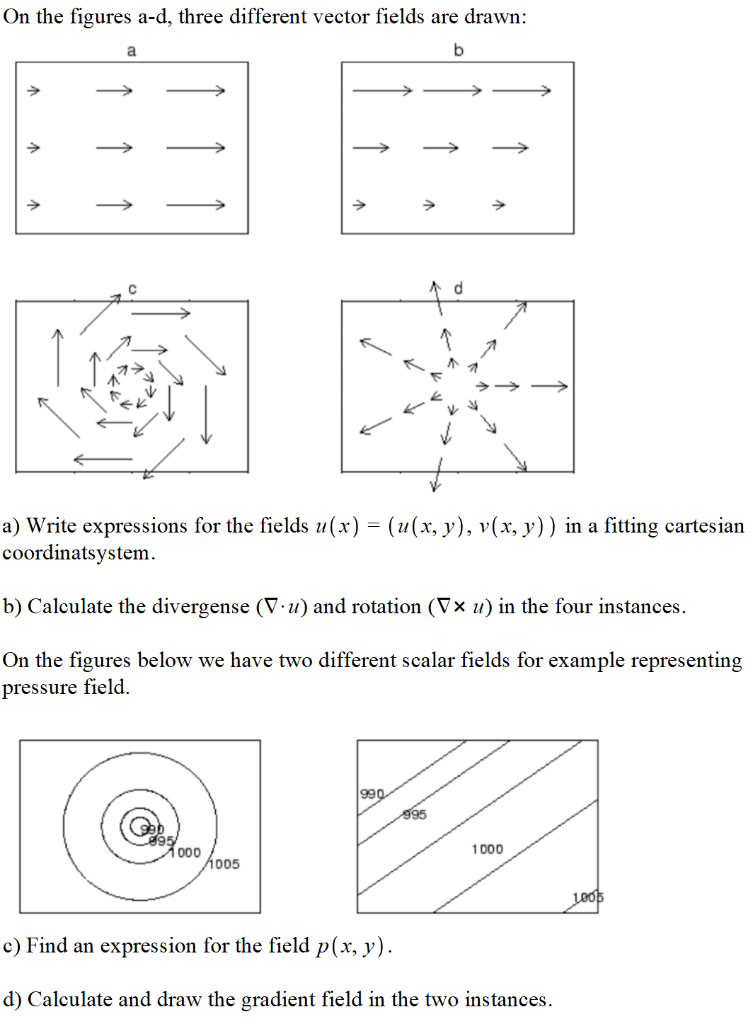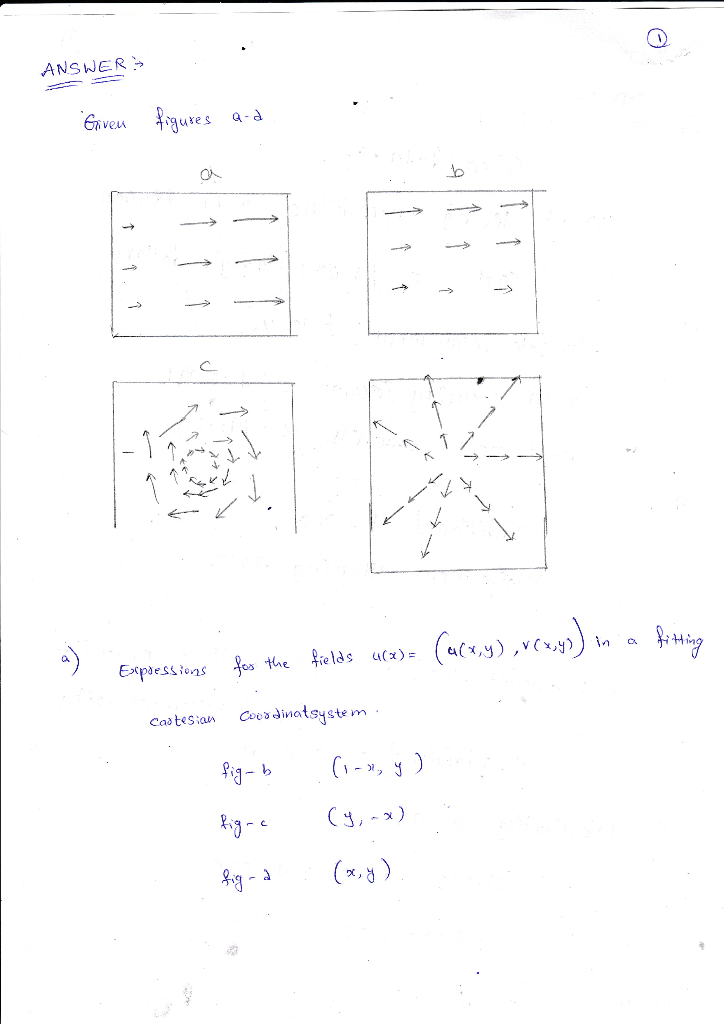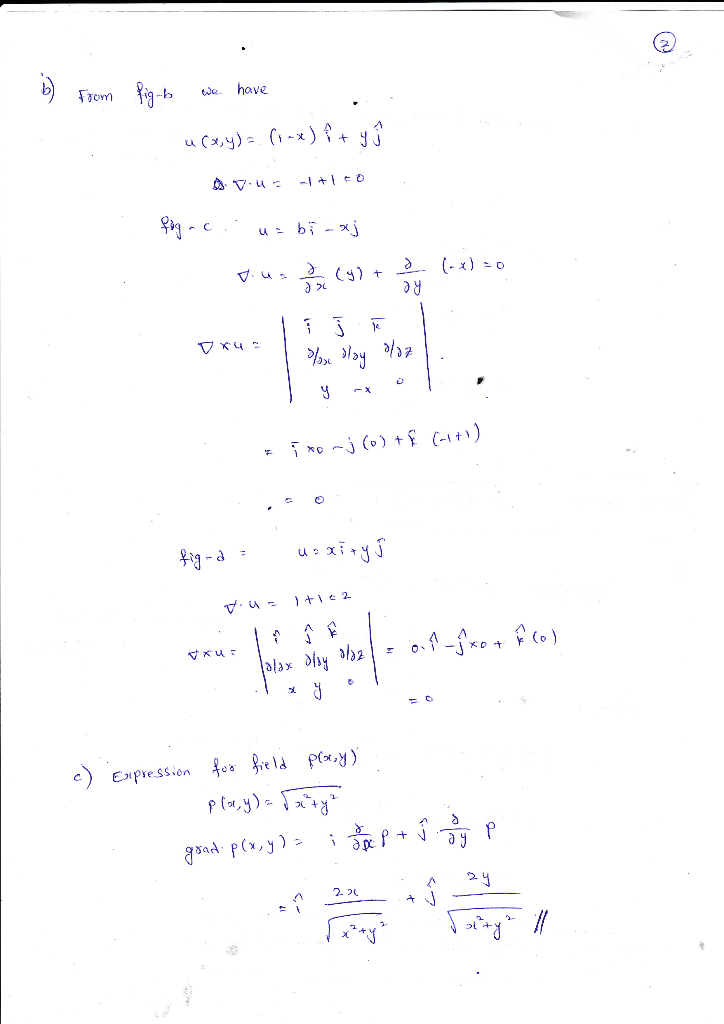Homework Help Question & Answers

# On the figures a-d, three different vector fields are drawn ラ a) Write expressions for the field...On the figures a-d, three different vector fields are drawn ラ a) Write expressions for the fields u(x)- (u(x, y), v(x, y)) in a fitting cartesian coordinatsystem b) Calculate the divergense (V-u) and rotation (Vx u) in the four instances. On the figures below we have two different scalar fields for example representing pressure field. 1000 005 c) Find an expression for the field p(x, y) d) Calculate and draw the gradient field in the two instances##### Add Answer of: On the figures a-d, three different vector fields are drawn ラ a) Write expressions for the field...
More Homework Help Questions Additional questions in this topic.

• #### New York’s MEGAMILLIONS lottery, a player wins 1st prize by selecting the correct 5 number combination when 5 different numbers from 1 through 56 are drawn, then selecting the correct MEGABALL number drawn from numbers 1 through 40

Need Online Homework Help?

Get FREE EXPERT Answers
WITHIN MINUTES
Related Questions